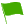Real & Rational Numbers (SHSAT Level Questions):

The difference between real and rational numbers is often confused by students. Remember rational and irrational numbers are part of the set of real numbers. Real numbers can be placed on a number line. Rational numbers are those real numbers that can be expressed as a fraction of integers in the form a/b. Irrational numbers are also real, but they cannot be expressed as a simple fraction of the form a/b.

1.Which of the following is a real number?
A.
B.
C.
D.

Question 1 of 5

2.The definition of a rational number is which of the following?
A.
B.
C.
D.

Question 2 of 5

3.An infinitely repeating decimal must also be which of the following?
A.
B.
C.
D.
E.

Question 3 of 5

4.All of the following are examples of real numbers except?
A.
B.
C.
D.

Question 4 of 5

5.The sum or product of a rational number and an irrational number must be which of the following?
A.
B.
C.
D.
E.

Question 5 of 5